Courses

# Asymptotic Worst Case Time & Space Complexity MCQ - 3

## 20 Questions MCQ Test GATE Computer Science Engineering(CSE) 2022 Mock Test Series | Asymptotic Worst Case Time & Space Complexity MCQ - 3

Description
This mock test of Asymptotic Worst Case Time & Space Complexity MCQ - 3 for Computer Science Engineering (CSE) helps you for every Computer Science Engineering (CSE) entrance exam. This contains 20 Multiple Choice Questions for Computer Science Engineering (CSE) Asymptotic Worst Case Time & Space Complexity MCQ - 3 (mcq) to study with solutions a complete question bank. The solved questions answers in this Asymptotic Worst Case Time & Space Complexity MCQ - 3 quiz give you a good mix of easy questions and tough questions. Computer Science Engineering (CSE) students definitely take this Asymptotic Worst Case Time & Space Complexity MCQ - 3 exercise for a better result in the exam. You can find other Asymptotic Worst Case Time & Space Complexity MCQ - 3 extra questions, long questions & short questions for Computer Science Engineering (CSE) on EduRev as well by searching above.
QUESTION: 1

### Consider the following C-function: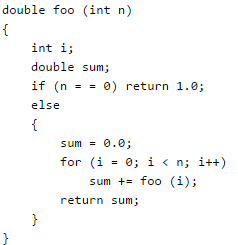Suppose we modify the above function foo() and store the values of foo (i), 0 < = i < n, as and when they are computed. With this modification, the time complexity for function foo() is significantly reduced. The space complexity of the modified function would be:

Solution:

Space complexity now is also O(n). We would need an array of size O(n). The space required for recursive calls would be O(1) as the values would be taken from stored array rather than making function calls again and again.

QUESTION: 2

### Two matrices M1 and M2 are to be stored in arrays A and B respectively. Each array can be stored either in row-major or column-major order in contiguous memory locations. The time complexity of an algorithm to compute M1 × M2 will be

Solution:

This is a trick question. Note that the questions asks about time complexity, not time taken by the program. for time complexity, it doesn't matter how we store array elements, we always need to access same number of elements of M1 and M2 to multiply the matrices. It is always constant or O(1) time to do element access in arrays, the constants may differ for different schemes, but not the time complexity.

QUESTION: 3

### Let A[1, ..., n] be an array storing a bit (1 or 0) at each location, and f(m) is a unction whose time complexity is θ(m). Consider the following program fragment written in a C like language: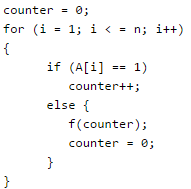Q. The complexity of this program fragment is

Solution:

Please note that inside the else condition, f() is called first, then counter is set to 0. Consider the following cases: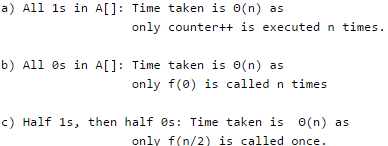QUESTION: 4

The recurrence equation

T(1) = 1
T(n) = 2T(n - 1) + n, n ≥ 2

evaluates to

Solution: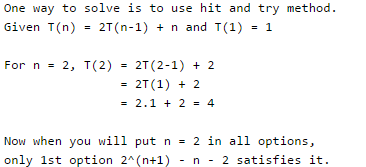QUESTION: 5

Consider the following three claims

1. (n + k)m = Θ(nm), where k and m are constants
2. 2n + 1 = O(2n)
3. 22n + 1 = O(2n)

Q. Which of these claims are correct ?

Solution:

(n + k)m and Θ(nm) are asymptotically same as theta notation can always be written by taking the leading order term in a polynomial expression. 2n + 1 and O(2n) are also asymptotically same as 2n + 1 can be written as 2 * 2n and constant multiplication/addition doesn't matter in theta notation. 22n + 1 and O(2n) are not same as constant is in power. See Asymptotic Notations for more details.

QUESTION: 6

In a permutation a1.....an of n distinct integers, an inversion is a pair (ai, aj) such that i < j and ai > aj. What would be the worst case time complexity of the Insertion Sort algorithm, if the inputs are restricted to permutations of 1.....n with at most n inversions?

Solution:

Insertion sort runs in Θ(n + f(n)) time, where f(n) denotes the number of inversion initially present in the array being sorted.

QUESTION: 7

Randomized quicksort is an extension of quicksort where the pivot is chosen randomly. What is the worst case complexity of sorting n numbers using randomized quicksort?

Solution:

Randomized quicksort has expected time complexity as O(nLogn), but worst case time complexity remains same. In worst case the randomized function can pick the index of corner element every time.

QUESTION: 8

The increasing order of following functions in terms of asymptotic complexity is: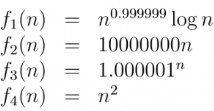Solution:
QUESTION: 9

Which one of the following is the recurrence equation for the worst case time complexity of the Quicksort algorithm for sorting n(≥ 2) numbers? In the recurrence equations given in the options below, c is a constant.

Solution:

In worst case, the chosen pivot is always placed at a corner position and recursive call is made for following.
a) for subarray on left of pivot which is of size n-1 in worst case.
b) for subarray on right of pivot which is of size 0 in worst case.

QUESTION: 10

Consider the following C function.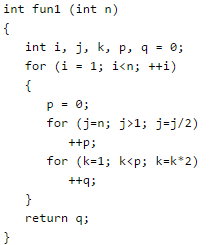Q. Which one of the following most closely approximates the return value of the function fun1?

Solution: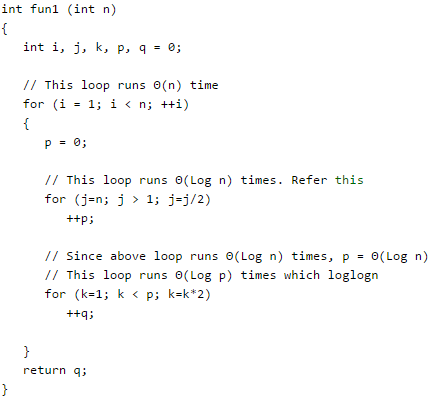T(n) = n(logn + loglogn) T(n) = n(logn) dominant But please note here we are return q which lies in loglogn so ans should be T(n) = nloglogn Refer this for details.

QUESTION: 11

An unordered list contains n distinct elements. The number of comparisons to find an element in this list that is neither maximum nor minimum is

Solution:

We only need to consider any 3 elements and compare them. So the number of comparisons is constants, that makes time complexity as Θ(1) The catch here is, we need to return any element that is neither maximum not minimum. Let us take an array {10, 20, 15, 7, 90}. Output can be 10 or 15 or 20 Pick any three elements from given liar. Let the three elements be 10, 20 and 7. Using 3 comparisons, we can find that the middle element is 10.

QUESTION: 12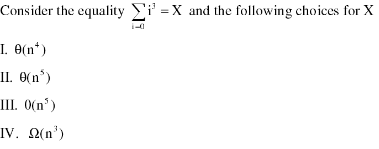Q. The equality above remains correct if X is replace by

Solution:

X = Sum of the cubes of {1, 2, 3, .. n| X = n2 (n+1)2 / 4

QUESTION: 13

In the following table, the left column contains the names of standard graph algorithms and the right column contains the time complexities of the algorithms. Match each algorithm with its time complexity.

1. Bellman-Ford algorithm
2. Kruskal’s algorithm
3. Floyd-Warshall algorithm
4. Topological sorting

A : O(m log n)
B : O(n3)
C : O(nm)
D : O(n + m)

Solution:
• Bellman-Ford algorithm: Time complexity: O(VE)
• Kruskal’s algorithm:Time Complexity: O(ElogE) or O(ElogV). Sorting of edges takes O(ELogE) time. After sorting, we iterate through all edges and apply find-union algorithm. The find and union operations can take atmost O(LogV) time. So overall complexity is O(ELogE + ELogV) time. The value of E can be atmost V^2, so O(LogV) are O(LogE) same. Therefore, overall time complexity is O(ElogE) or O(ElogV)
• Floyd-Warshall algorithmTime Complexity: O(V^3)
• Topological sortingTime Complexity: The above algorithm is simply DFS with an extra stack. So time complexity is same as DFS which is O(V+E).
QUESTION: 14

Let T(n) be a function defined by the recurrence T(n) = 2T(n/2) + √n for n ≥ 2 and T(1) = 1 Which of the following statements is TRUE?

Solution:

n(logba) = n which is = n^(1-.5) = O(sqrt n) then by applying case 1 of master method we get T(n) = Θ(n)

QUESTION: 15

The worst case running times of Insertion sort, Merge sort and Quick sort, respectively, are:

Solution:
• Insertion Sort takes Θ(n2) in worst case as we need to run two loops. The outer loop is needed to one by one pick an element to be inserted at right position. Inner loop is used for two things, to find position of the element to be inserted and moving all sorted greater elements one position ahead. Therefore the worst case recursive formula is T(n) = T(n-1) + Θ(n).
• Merge Sort takes Θ(n Log n) time in all cases. We always divide array in two halves, sort the two halves and merge them. The recursive formula is T(n) = 2T(n/2) + Θ(n).
• QuickSort takes Θ(n2) in worst case. In QuickSort, we take an element as pivot and partition the array around it. In worst case, the picked element is always a corner element and recursive formula becomes T(n) = T(n-1) + Θ(n). An example scenario when worst case happens is, arrays is sorted and our code always picks a corner element as pivot.
QUESTION: 16

Assume that the algorithms considered here sort the input sequences in ascending order. If the input is already in ascending order, which of the following are TRUE ?

I. Quicksort runs in Θ(n2) time
II. Bubblesort runs in Θ(n2) time
III. Mergesort runs in Θ(n) time
IV. Insertion sort runs in Θ(n) time

Solution:

I. Given an array in ascending order, Recurrence relation for total number of comparisons for quicksort will be T(n) = T(n-1)+O(n) //partition algo will take O(n) comparisons in any case. = O(n^2)
II. Bubble Sort runs in Θ(n^2) time If an array is in ascending order, we could make a small modification in Bubble Sort Inner for loop which is responsible for bubbling the kth largest element to the end in kth iteration. Whenever there is no swap after the completion of inner for loop of bubble sort in any iteration, we can declare that array is sorted in case of Bubble Sort taking O(n) time in Best Case.
III. Merge Sort runs in Θ(n) time Merge Sort relies on Divide and Conquer paradigm to sort an array and there is no such worst or best case input for merge sort. For any sequence, Time complexity will be given by following recurrence relation, T(n) = 2T(n/2) + Θ(n) // In-Place Merge algorithm will take Θ(n) due to copying an entire array. = Θ(nlogn)
IV. Insertion sort runs in Θ(n) time Whenever a new element which will be greater than all the elements of the intermediate sorted sub-array (because given array is sorted) is added, there won't be any swap but a single comparison. In n-1 passes we will be having 0 swaps and n-1 comparisons. Total time complexity = O(n) // N-1 Comparisons

//// For an array already sorted in ascending order, Quicksort has a complexity Θ(n2) [Worst Case] Bubblesort has a complexity Θ(n) [Best Case] Mergesort has a complexity Θ(n log n) [Any Case] Insertsort has a complexity Θ(n) [Best Case]

QUESTION: 17

A problem in NP is NP-complete if

Solution:

A problem in NP becomes NPC if all NP problems can be reduced to it in polynomial time. This is same as reducing any of the NPC problem to it. 3-SAT being an NPC problem, reducing it to a NP problem would mean that NP problem is NPC.

QUESTION: 18

The characters a to h have the set of frequencies based on the first 8 Fibonacci numbers as follows

a : 1, b : 1, c : 2, d : 3, e : 5, f : 8, g : 13, h : 21

A Huffman code is used to represent the characters. What is the sequence of characters corresponding to the following code? 110111100111010

Solution:

Background Required -  Generating Prefix codes using Huffman Coding. First we apply greedy algorithm on the frequencies of the characters to generate the binary tree as shown in the Figure given below. Assigning 0 to the left edge and 1 to the right edge, prefix codes for the characters are as below.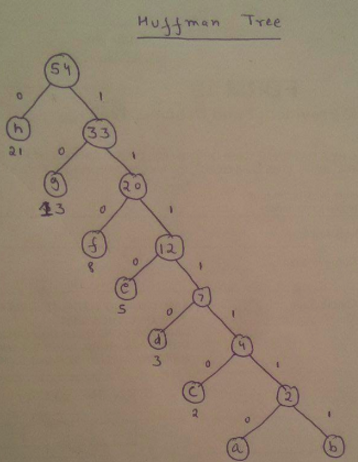a - 1111110
b - 1111111
c - 111110
d - 11110
e - 1110
f - 110
g - 10
h - 0
Given String can be decomposed as 110 11110 0 1110 10 fdheg

QUESTION: 19

What is the size of the smallest MIS(Maximal Independent Set) of a chain of nine nodes?

Solution:

A set of vertices is called independent set such that no two vertices in the set are adjacent. A maximal independent set (MIS) is an independent set which is not subset of any other independent set. The question is about smallest MIS. We can see in below diagram, the three highlighted vertices (2nd, 5th and 8th) form a maximal independent set (not subset of any other MIS) and smallest MIS.

0----0----0----0----0----0----0----0----0

QUESTION: 20

Arrange the following functions in increasing asymptotic order:
A. n1/3
B. en
C. n7/4
D. n log9
E. 1.0000001n

Solution: